#### 期刊菜单

Percolation Characteristics of Oil-Water Two-Phase Fluids in Micro Channels
DOI: 10.12677/APF.2018.83007, PDF, HTML, XML, 下载: 965  浏览: 2,484  国家自然科学基金支持

Abstract: The flow in micro channels is the microscopic foundation of the flow in porous media. Based on the flow in micro channels, the percolation unit model was developed for the oil-water two-phase flow in a porous medium. This model indicates that the relative permeabilities of oil-water two-phase flow in a porous medium are related to the phase geometry distribution and fluid saturation in micro channels, but have nothing to do with the porosity of the porous medium. An experimental device is designed in which the micro channels are fabricated by microfluidic technology. The percolation characteristics of two-phase flow in the micro channels are experimentally investigated in such device with immiscible oil and water as fluids. The experimental data show that the relative permeability curves of oil-water two-phase flow are approximately the same for the micro channels with similar geometry sizes, but obviously different for the micro channels with distinct geometry sizes. In addition to the fluid saturation and the pressure drop, the total volume flow rate is discovered to be a parameter affecting the relative permeability of oil-water two-phase flow. The experimental results are consistent with the inferences drawn from the percolation unit model developed.

1. 引言

2. 渗流单元模型

2.1. 渗流单元与绝对渗透率

${J}_{i}=\varphi {u}_{ip}$ (1)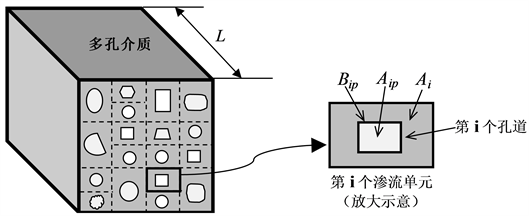Figure 1. Percolation unit model

$\frac{\Delta {p}_{f}}{L}=\frac{1}{8}{\lambda }_{ip}\rho {u}_{ip}^{2}\frac{{B}_{ip}}{{A}_{ip}}$ (2)

${\lambda }_{ip}=\frac{64}{R{e}_{ip}}=\frac{64\mu }{{u}_{ip}\rho {d}_{ip}}$ (3)

${d}_{ip}=\frac{4{A}_{ip}}{{B}_{ip}}=\frac{4\varphi {A}_{i}}{{B}_{ip}}$ (4)

${A}_{ip}=\varphi {A}_{i}$ (5)

${J}_{i}=\frac{{\varphi }^{3}{A}_{i}^{2}}{2{B}_{ip}^{2}}\frac{1}{\mu }\frac{\Delta {p}_{f}}{L}$ (6)

${J}_{i}=\frac{{k}_{i}}{\mu }\frac{\Delta {p}_{f}}{L}$ (7)

${k}_{i}=\frac{1}{2}\frac{{\varphi }^{3}{A}_{i}^{2}}{{B}_{ip}^{2}}$ (8)

$J=\frac{\underset{i=1}{\overset{n}{\sum }}{J}_{i}{A}_{i}}{\underset{i=1}{\overset{n}{\sum }}{A}_{i}}=\frac{1}{\mu }\frac{\Delta {p}_{f}}{L}\frac{\underset{i=1}{\overset{n}{\sum }}\frac{1}{2}{\varphi }^{3}\frac{{A}_{i}^{3}}{{B}_{ip}^{2}}}{\underset{i=1}{\overset{n}{\sum }}{A}_{i}}$ (9)

$k=\text{=}\frac{\underset{i=1}{\overset{n}{\sum }}{k}_{i}{A}_{i}}{\underset{i=1}{\overset{n}{\sum }}{A}_{i}}$ (10)

2.2. 两相渗流的相对渗透率

$\varphi {A}_{i}{S}_{ipw}\frac{\text{d}{p}_{w}}{\text{d}x}+\frac{1}{8}{\lambda }_{ipw}{\rho }_{w}{u}_{ipw}^{2}{B}_{ipw}+\varphi {A}_{i}{S}_{ipw}{\rho }_{w}g\mathrm{sin}\theta =0$ (11)

$\varphi {A}_{i}{S}_{ipo}\frac{\text{d}{p}_{o}}{\text{d}x}+\frac{1}{8}{\lambda }_{ipo}{\rho }_{o}{u}_{ipo}^{2}{B}_{ipo}+\varphi {A}_{i}{S}_{ipo}{\rho }_{o}g\mathrm{sin}\theta =0$ (12)

${J}_{iw}=\varphi {S}_{ipw}{u}_{ipw}=\varphi \frac{{Q}_{ipw}}{{A}_{ip}}$ (13)

${J}_{io}=\varphi {S}_{ipo}{u}_{ipo}=\varphi \frac{{Q}_{ipo}}{{A}_{ip}}$ (14)

${\lambda }_{ipw}=\frac{64}{R{e}_{ipw}}=\frac{64{\mu }_{w}}{{u}_{ipw}{\rho }_{w}{d}_{ipw}}$ (15)

${\lambda }_{ipo}=\frac{64}{R{e}_{ipo}}=\frac{64{\mu }_{o}}{{u}_{ipo}{\rho }_{o}{d}_{ipo}}$ (16)

${d}_{ipw}=\frac{4{A}_{i}\varphi {S}_{ipw}}{{B}_{ipw}}$ (17)

${d}_{ipo}=\frac{4{A}_{i}\varphi {S}_{ipo}}{{B}_{ipo}}$ (18)

$\frac{\text{d}{p}_{w}}{\text{d}x}=-\frac{\Delta {p}_{w}}{L}$ (19)

$\frac{\text{d}{p}_{o}}{\text{d}x}=-\frac{\Delta {p}_{o}}{L}$ (20)

${J}_{iw}=\frac{{\varphi }^{3}{A}_{i}^{2}{S}_{ipw}^{3}}{2{B}_{ipw}^{2}}\cdot \frac{1}{{\mu }_{w}}\left(\frac{\Delta {p}_{w}}{L}+{\rho }_{w}g\mathrm{sin}\theta \right)$ (21)

${J}_{io}=\frac{{\varphi }^{3}{A}_{i}^{2}{S}_{ipo}^{3}}{2{B}_{ipo}^{2}}\cdot \frac{1}{{\mu }_{o}}\left(\frac{\Delta {p}_{o}}{L}+{\rho }_{o}g\mathrm{sin}\theta \right)$ (22)

${J}_{iw}=\frac{{k}_{irw}{k}_{i}}{{\mu }_{w}}\left(\frac{\Delta p}{L}+{\rho }_{w}g\mathrm{sin}\theta \right)$ (23)

${J}_{io}=\frac{{k}_{iro}{k}_{i}}{{\mu }_{o}}\left(\frac{\Delta p}{L}+{\rho }_{o}g\mathrm{sin}\theta \right)$ (24)

${k}_{irw}={S}_{ipw}^{3}{\left(\frac{{B}_{ip}}{{B}_{ipw}}\right)}^{2}$ (25)

${k}_{iro}={S}_{ipo}^{3}{\left(\frac{{B}_{ip}}{{B}_{ipo}}\right)}^{2}$ (26)

${J}_{w}=\frac{\underset{i=1}{\overset{n}{\sum }}{J}_{iw}{A}_{i}}{\underset{i=1}{\overset{n}{\sum }}{A}_{i}}=\frac{1}{{\mu }_{w}}\left(\frac{\Delta p}{L}+{\rho }_{w}g\mathrm{sin}\theta \right)\frac{\underset{i=1}{\overset{n}{\sum }}{k}_{irw}{k}_{i}{A}_{i}}{\underset{i=1}{\overset{n}{\sum }}{A}_{i}}$ (27)

${J}_{o}=\frac{\underset{i=1}{\overset{n}{\sum }}{J}_{io}{A}_{i}}{\underset{i=1}{\overset{n}{\sum }}{A}_{i}}=\frac{1}{{\mu }_{o}}\left(\frac{\Delta p}{L}+{\rho }_{o}g\mathrm{sin}\theta \right)\frac{\underset{i=1}{\overset{n}{\sum }}{k}_{iro}{k}_{i}{A}_{i}}{\underset{i=1}{\overset{n}{\sum }}{A}_{i}}$ (28)

${J}_{w}=\frac{{k}_{rw}k}{{\mu }_{w}}\left(\frac{\Delta p}{L}+{\rho }_{w}g\mathrm{sin}\theta \right)$ (29)

${J}_{o}=\frac{{k}_{ro}k}{{\mu }_{o}}\left(\frac{\Delta p}{L}+{\rho }_{o}g\mathrm{sin}\theta \right)$ (30)

${k}_{rw}k=\frac{\underset{i=1}{\overset{n}{\sum }}{k}_{irw}{k}_{i}{A}_{i}}{\underset{i=1}{\overset{n}{\sum }}{A}_{i}}$ (31)

${k}_{ro}k=\frac{\underset{i=1}{\overset{n}{\sum }}{k}_{iro}{k}_{i}{A}_{i}}{\underset{i=1}{\overset{n}{\sum }}{A}_{i}}$ (32)

3. 两相渗流的微流动实验

${Q}_{ipo}={\beta }_{o}Q$ (33)

${Q}_{ipw}={\beta }_{w}Q=\left(1-{\beta }_{o}\right)Q$ (34)Table 1. Geometry of microchannels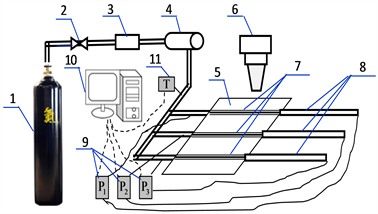1——氮气瓶，2——减压阀，3——压力定值器，4——油水乳化液储液器，5——硅片，6——显微镜，7——微通道，8——体积管流量计，9——差压变送器，10——计算机，11——温度传感器

Figure 2. The experimental device of micro flow

4. 结果与讨论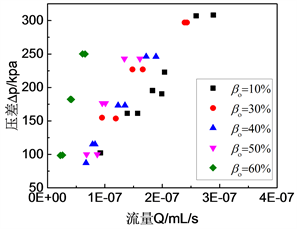(a)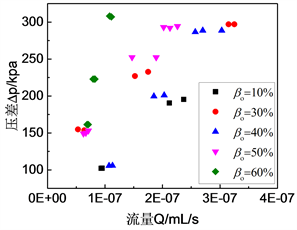(b)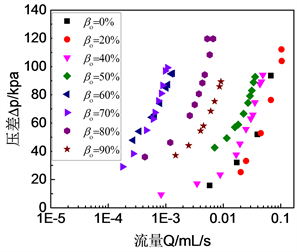(c)

Figure 3. Change of flow rate with differential pressure and oil volume fraction. (a) Pore 1; (b) Pore 2; (c) Pore 3

${k}_{irw}=\frac{2\left(1-{\beta }_{o}\right)Q{\mu }_{w}{B}_{ip}^{2}}{{A}_{ip}^{3}\frac{\Delta p}{L}}$ (35)

${k}_{iro}=\frac{2{\beta }_{o}Q{\mu }_{o}{B}_{ip}^{2}}{{A}_{ip}^{3}\frac{\Delta p}{L}}$ (36)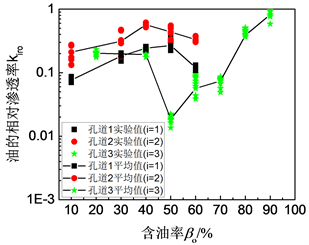(a)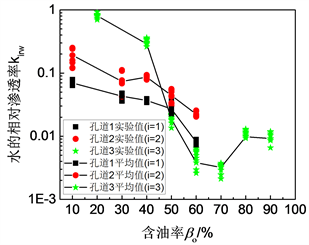(b)

Figure 4. The curve of relative permeability and oil content

5. 结论

1) 油水两相的相对渗透率与多孔介质的孔隙度无关，只依赖于微通道中相态分布的几何尺度以及流体饱和度。

2) 对于相同尺度等级的微通道，油水两相渗流的平均相对渗透率曲线类似；对于尺度等级明显不同的微通道，其油水两相渗流的平均相对渗透率曲线有显著差异。

3) 油水两相的相对渗透率曲线之所以存在差异，是由于相对渗透率不仅与油水两相的饱和度有关，还与其湿周等参数有关。对应于相同的含油率或饱和度，油水两相在不同尺度等级的微通道会有不同的湿周，在相同尺度等级的微通道中会有近似的湿周，这是引起相对渗透率曲线存在差异的微观因素。

4) 实验结果与渗流单元模型都表明，即使层流状态下，油水两相的相对渗透率还与两相流体在微通道中的体积流量、压降等参数有关。

NOTES

*通讯作者。

  Denney, D. (2005) A Low-Permeability Case Study of 5 Years of Continuous Well-Performance Improvement. Journal of Petroleum Technology, 57, 41-42. https://doi.org/10.2118/0105-0041-JPT  Denney, D. (2004) Characterization of Water Injection in Low-Permeability Rock Using Sandstone Micromodels. Journal of Petroleum Technology, 56, 71-72. https://doi.org/10.2118/0504-0071-JPT  Cinar, Y. and Franklin, M. (2005) Measurement of Three-Phase Relative Permea-bility with IFT Variation. SPE Reservoir Evaluation and Engineering, 8, 33-43. https://doi.org/10.2118/89419-PA  杨悦, 周芳德. 低渗透复杂油藏渗流理论基础[M]. 西安: 西安交通大学出版社, 2010.  Alizadeh, A.H. and Piri, M. (2014) Three-Phase Flow in Porousmedia: A Review of Experimental Studies on Relative Permeability. Reviews of Geophysics, 52, 468-521. https://doi.org/10.1002/2013RG000433  Gunde, A., Babadagli, T., Roy, S.S. and Mitra, S.K. (2013) Pore-Scale Interfacial Dynamics and Oil-Water Relative Permeabilities of Capillary Driven Counter-Current Flow in Fractured Porous Media. Journal of Petroleum Science and Engineering, 103, 106-114. https://doi.org/10.1016/j.petrol.2013.02.005  Poate, J., Illangasekare, T., Kazemi, H. and Kee, R. (2015) Pore Scale Phenomena: Frontiers in Energy and Environment. World Scientific Publishing Co Pte Ltd., Singapore. https://doi.org/10.1142/9305  Mahmoudi, S., Mohammadzadeh, O., Hashemi, A. and Kord, S. (2017) Pore-Scale Numerical Modeling of Relative Permeability Curves for CO2-Oil Fluid System with an Application in Immiscible CO2 Flooding. Journal of Petroleum Exploration and Production Technology, 7, 235-249. https://doi.org/10.1007/s13202-016-0256-4  严宗毅. 低雷诺数流理论[M]. 北京: 北京大学出版社, 2002.  Marle, C.M. (1981) Multiphase Flow in Porous Media. Gulf Publishing Company, Houston.  邓英尔, 等. 高等渗流理论与方法[M]. 北京: 科学出版社, 2007.  Wallis, G.B. (1969) One-Dimensional Two-Phase Flow. McGraw-Hill Inc., New York.